# Overview of MATLAB Offline Analysis Tools

Read block files directly from disk or SEV files directly from RS4.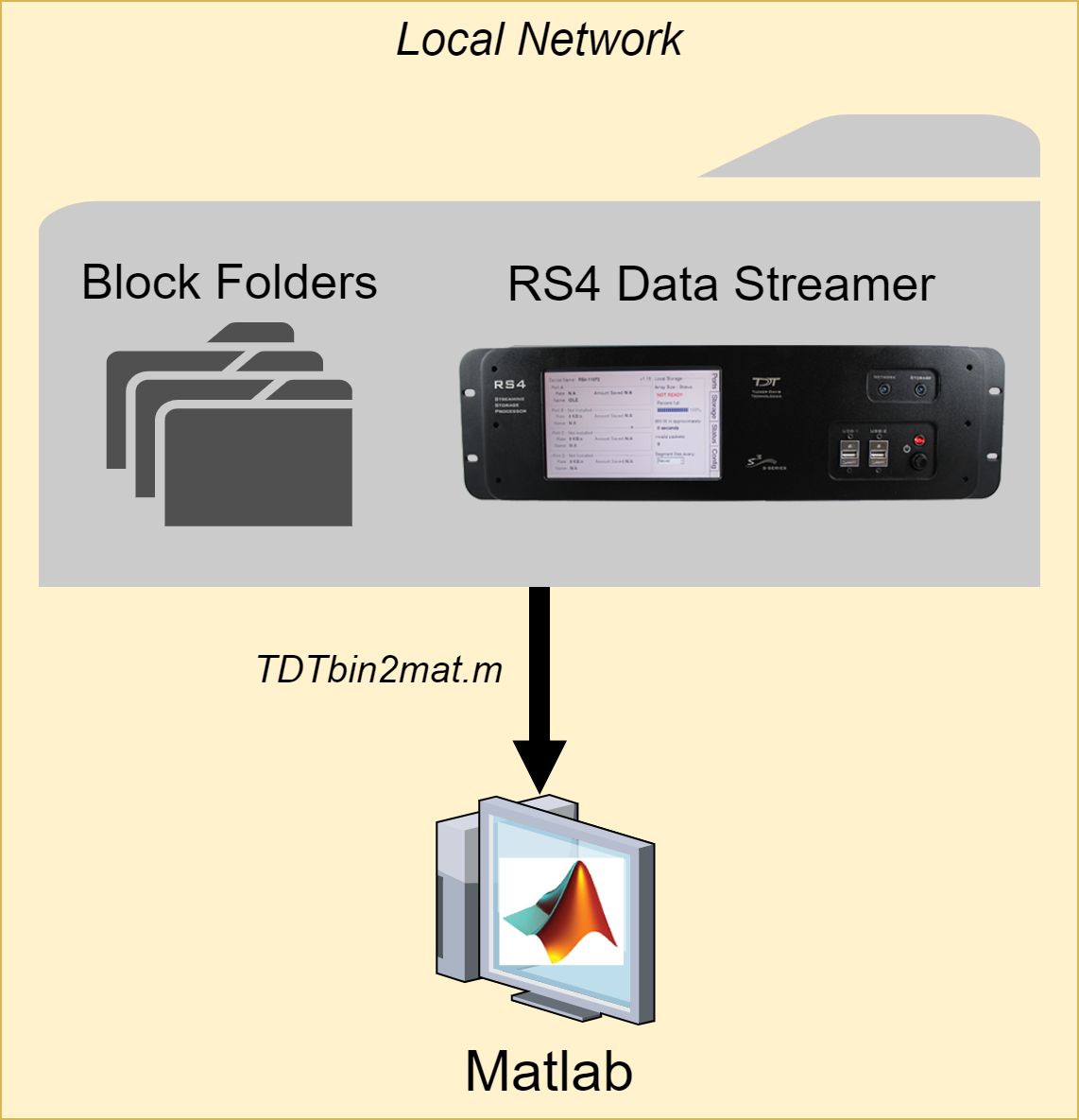See TDT Data Storage for a description of the folder structure.

## TDTbin2mat

`TDTbin2mat` is an all-in-one function for reading TDT data into MATLAB. It needs only one input: the block path.

``````data = TDTbin2mat('C:\TDT\Synapse\Tanks\Exp1-160921-120606\Sub1-1');
``````

`TDTbin2mat` will return a structure containing all recorded data from that block, organized by type. See TDT Data Types for a description of the data types.

`TDTbin2mat` uses parameter value combinations to refine the imported data. To extract specific event types only, use the `'TYPE'` option. For example, to import epocs and snippets only, use this:

``````data = TDTbin2mat('C:\TDT\TDTExampleData\Algernon-180308-130351', ...
'TYPE', {'epocs', 'snips'})
``````

Use the `'STORE'` option to extract a particular data store by name, in this example a streaming event called `'Wav1'`. Combine this with the `'CHANNEL'` option to extract a single channel, or list of channels, in this case channel 2:

``````data = TDTbin2mat('C:\TDT\TDTExampleData\Algernon-180308-130351', ...
'STORE', 'Wav1', 'CHANNEL', 2);
``````

You can also filter by time, if you are only interested in portions of the recording, or if the entire recording won't fit into available memory (RAM) at one time. Use the `'T1'` and `'T2'` options to specify the start and stop time, in seconds, to retrieve from the block. This example reads only from time T1=10s to time T2=20s of the block into MATLAB:

``````data = TDTbin2mat('C:\TDT\TDTExampleData\Algernon-180308-130351', ...
'T1', 10, 'T2', 20);
``````

`TDTbin2mat` offers many more useful options that are described in its help documentation.

``````>> help TDTbin2mat
``````

## TDTfilter

`TDTfilter` applies advanced epoc filtering to extracted data. For example, if you only want to look at data around a certain epoc event, you will use `TDTfilter` to do this. See the Raster/PSTH example for a complete demonstration.

To only look at data around the epoc event timestamps, use a `'TIME'` filter. In this example, data from 20 ms before the Levl epoc to 50ms after the onset is retained.

``````data = TDTfilter(data, 'Levl', 'TIME', [-0.02, 0.07]);
``````

To only look at data when an epoc was a certain value, use a `'VALUES'` filter. In this example, only data when the Freq epoc was equal to 9000 or 10000 is retained.

``````data = TDTfilter(data, 'Freq', 'VALUES', [9000, 10000]);
``````

If you want to look for a particular behavioral response that occurs sometime during the allowed time range, use the `'MODIFIERS'` filter. In this example, only data when the Freq epoc was 10000 AND the Resp epoc had a value of 1 sometime during the Freq epoc is retained.

``````data = TDTfilter(data, 'Freq', 'VALUES', );
data = TDTfilter(data, 'Resp', 'MODIFIERS', );
``````

As you can see, for complex filtering the output from one call to `TDTfilter` can become the input to the next call to `TDTfilter`. If your data sets are large, or if you are iterating through many combinations of epoc variables, it is preferred to extract only the epocs and do all of the epoc filtering first to find the valid time ranges that match the filter, and then use this as the `'RANGES'` input to `TDTbin2mat` to extract all events (including snips, streams) on only those valid time ranges.

``````% read just the epoc events
data = TDTbin2mat(block_path, 'TYPE', {'epocs'});

% use the epocs to find time ranges we want
data = TDTfilter(data, 'Freq', 'VALUES', [9000, 10000]);
data = TDTfilter(data, 'Levl', 'VALUES', [70, 80, 90]);
data = TDTfilter(data, 'Resp', 'MODIFIERS', );

% read just the value time ranges from the whole data set
data = TDTbin2mat(block_path, 'RANGES', data.time_ranges);
``````

## SEV2mat

`SEV2mat` reads SEV files into a MATLAB structure. SEV files are created by the RS4 Data Streamer or by enabling the Discrete Files option when streaming continuous signals in Synapse. SEV files consist of a single channel of data per file, with a short header, so it is very fast to read them. `TDTbin2mat` will automatically call `SEV2mat` if it finds SEV files in the block directory. Like `TDTbin2mat`, `SEV2mat` needs only one input: the block path.

``````data = SEV2mat('C:\TDT\Synapse\Tanks\Exp1-160921-120606\Sub1-1');
``````

`SEV2mat` will return a structure containing the streams that it found. Each stream field includes the data array and sampling rate.

## TDTfft

`TDTfft` performs frequency analysis on the data stream. It takes the stream data output of `TDTbin2mat` and a channel number as inputs. In this example, it is plotting channel 1 of the `'LFP1'` store from an example block.

``````data = TDTbin2mat('C:\TDT\TDTExampleData\Algernon-180308-130351');
TDTfft(data.streams.LFP1, 1);
``````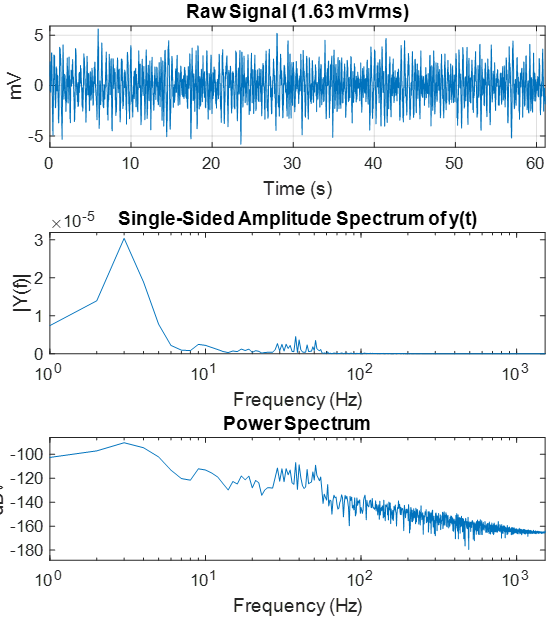Use the `'FREQ'` input to specify the frequency range that you're interested in.

``````% look at frequencies 0 Hz to 300 Hz only
TDTfft(data.streams.LFP1, 1, 'FREQ', [0, 300]);
``````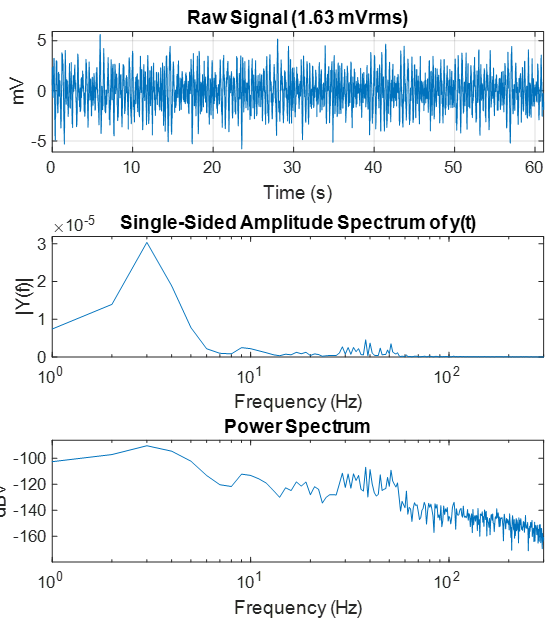Often the frequency plot is noisy. Use the `'NUMAVG'` input to smooth out the frequency plot and better isolate the components with the most power through the entire recording. This breaks the data into chunks, performs the FFT on those chunks, and then plots the average.

``````% split data up into 20 chunks and plot average FFT
TDTfft(data.streams.LFP1, 1, 'FREQ', [0, 300], 'NUMAVG', 20);
``````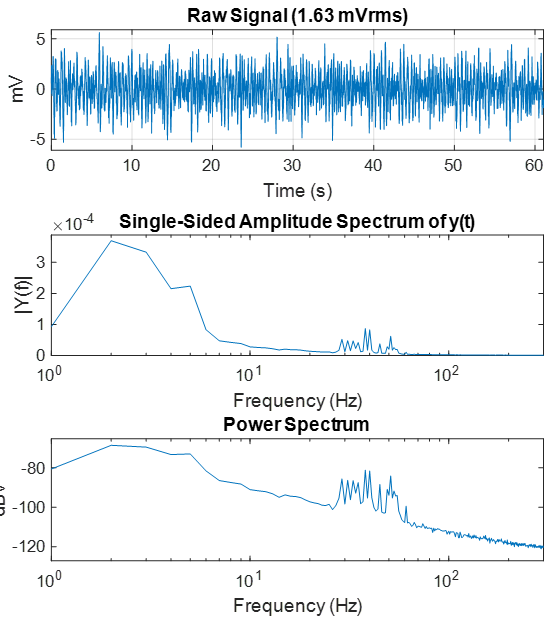Set the `'SPECPLOT'` input to 1 to also display a spectrogram of the entire data stream. If the data set is too long then MATLAB could run out of memory to do this, and you would need to use the `T1` and `T2` parameters in `TDTbin2mat` to look at smaller chunks of data.

``````data = TDTbin2mat('C:\TDT\TDTExampleData\Algernon-180308-130351', 'T2', 10);
TDTfft(data.streams.LFP1, 1, 'FREQ', [0, 100], 'NUMAVG', 20,'SPECPLOT', 1);
``````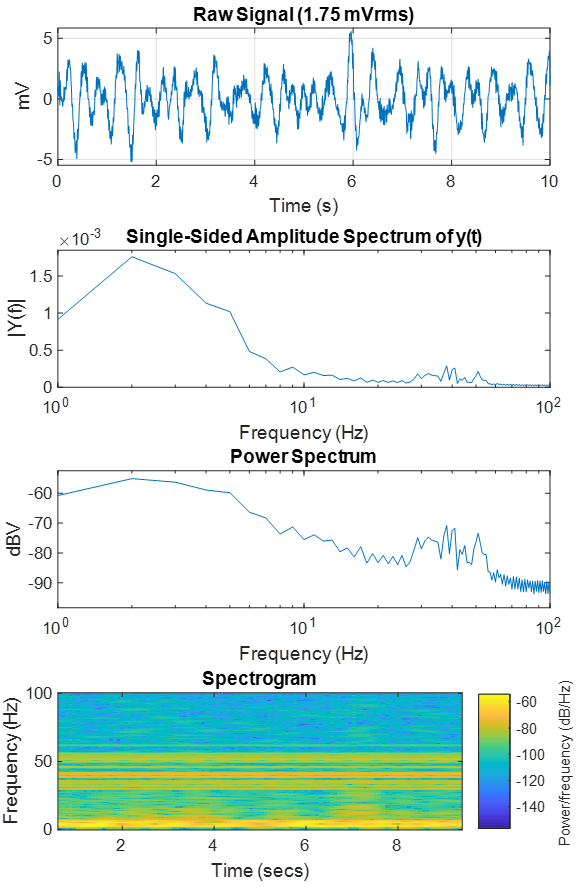See the LFP plot example for a full demonstration.

## TDTdigitalfilter

`TDTdigitalfilter` mimics the hardware digital filters in the Neural Stream Processor gizmo. It takes the output of `TDTbin2mat` and applies the specified digital filter to the specified streaming data store. Here is a simple DC-20 Hz bandpass filter example:

``````data = TDTbin2mat('C:\TDT\TDTExampleData\Algernon-180308-130351', 'T2', 10);
subplot(2,1,1);
plot(data.streams.Wav1.data(1,:));
data = TDTdigitalfilter(data, 'Wav1', [0, 20]); % look at 0-20 Hz only
subplot(2,1,2);
plot(data.streams.Wav1.data(1,:));
``````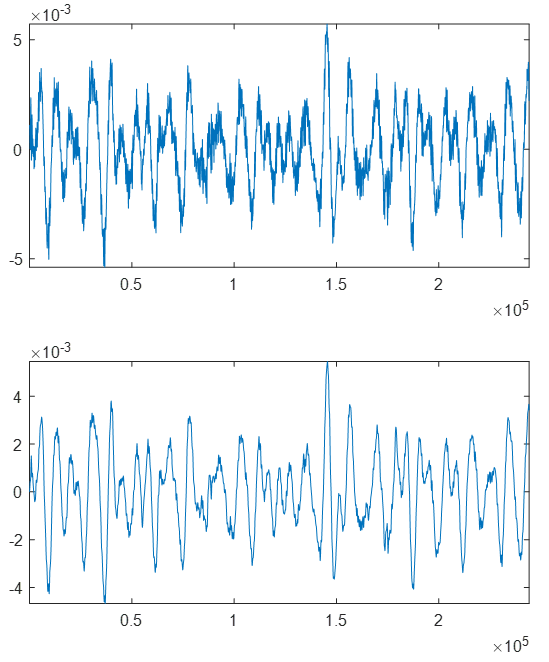Use the `'ORDER'` input to set the filter order. By default it is a 2 for a second order filter.

``````data = TDTdigitalfilter(data, 'Wav1', [0, 20], 'ORDER', 8);
``````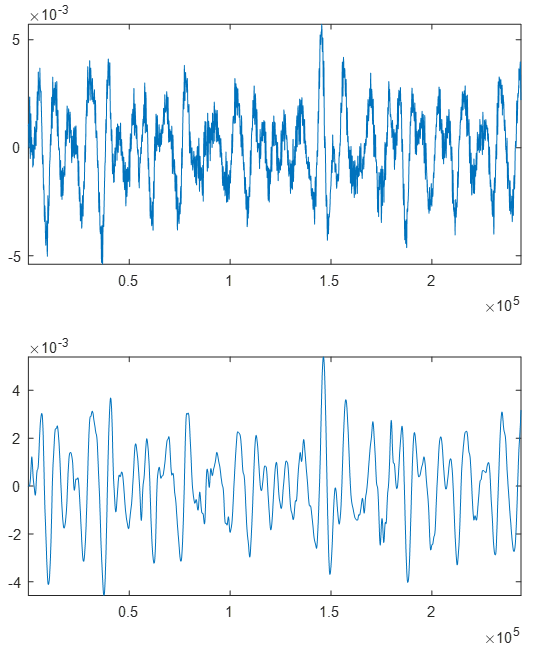You can also add a notch filter.

``````data = TDTdigitalfilter(data, 'Wav1', 'NOTCH', 60, 'ORDER', 4);
``````

## TDTthresh

`TDTthresh` takes continuous data and applies a thresholding algorithm to extract snippets. It has two modes, `'manual'` and `'auto'`. In `'manual'` mode, supply the absolute threshold input `'THRESH'` to extract snippets. Can be negative for negative-first spike detection.

In `'auto'` mode, a multiple of the sliding RMS window is used to calculate the instantaneous threshold. Set the `'TAU'` parameter to specify the time window, and set the `'STD'` parameter to set the scalar. So if TAU=5 and STD=6, then the threshold will track 6*RMS of the previous 5 seconds.

The new snippet store generated from `TDTthresh` is called `'Snip'`.

``````data = TDTbin2mat('C:\TDT\TDTExampleData\Algernon-180308-130351', 'T2', 10);
data = TDTdigitalfilter(data, 'Wav1', [300 5000]);
data = TDTthresh(data, 'Wav1', 'MODE', 'auto');
subplot(2,1,1);
plot(data.streams.Wav1.data(1,:));
subplot(2,1,2);
plot(data.snips.Snip.data');
``````

You can set the `'TETRODE'` flag to extract snippets organized by groups of 4 channels. If the threshold crosses on one channel, all 4 channels will get a snippet.

`TDTthresh` has a few more customizations you can explore in its help documentation.

``````>> help TDTthresh
``````

See the Rethreshold Example for a complete demonstration of `TDTdigitalfilter` and `TDTthresh`.